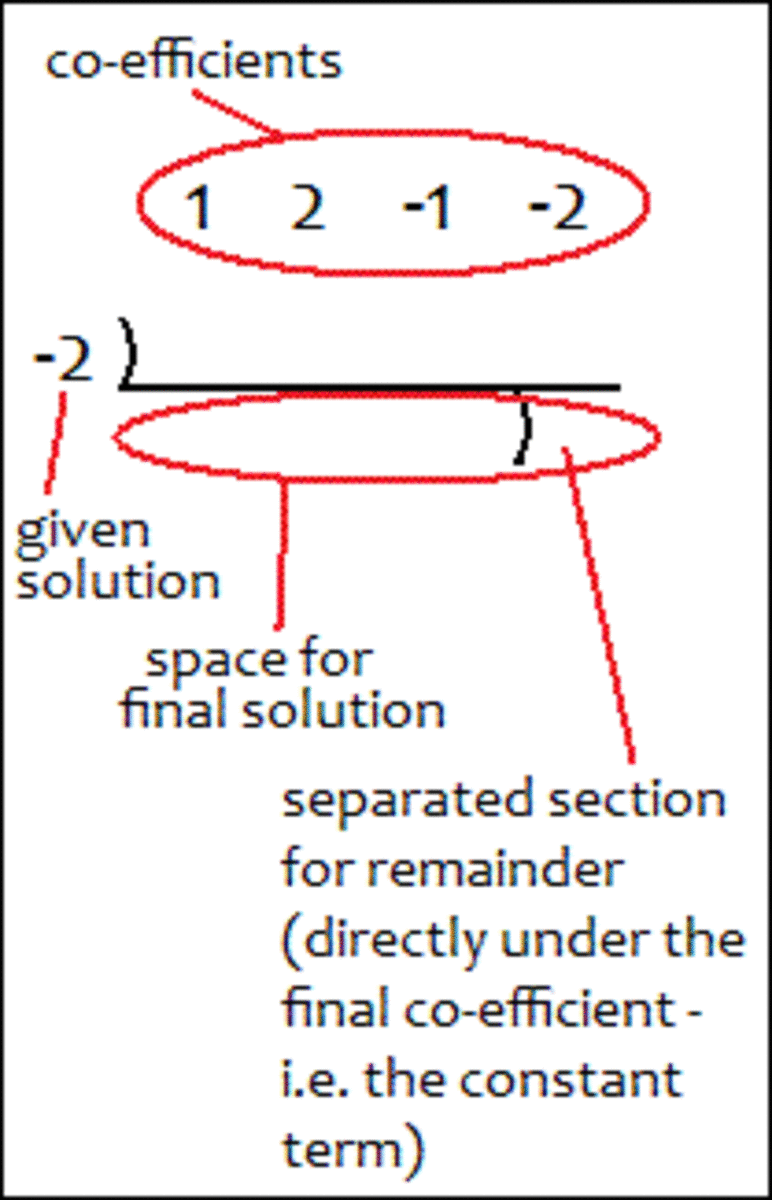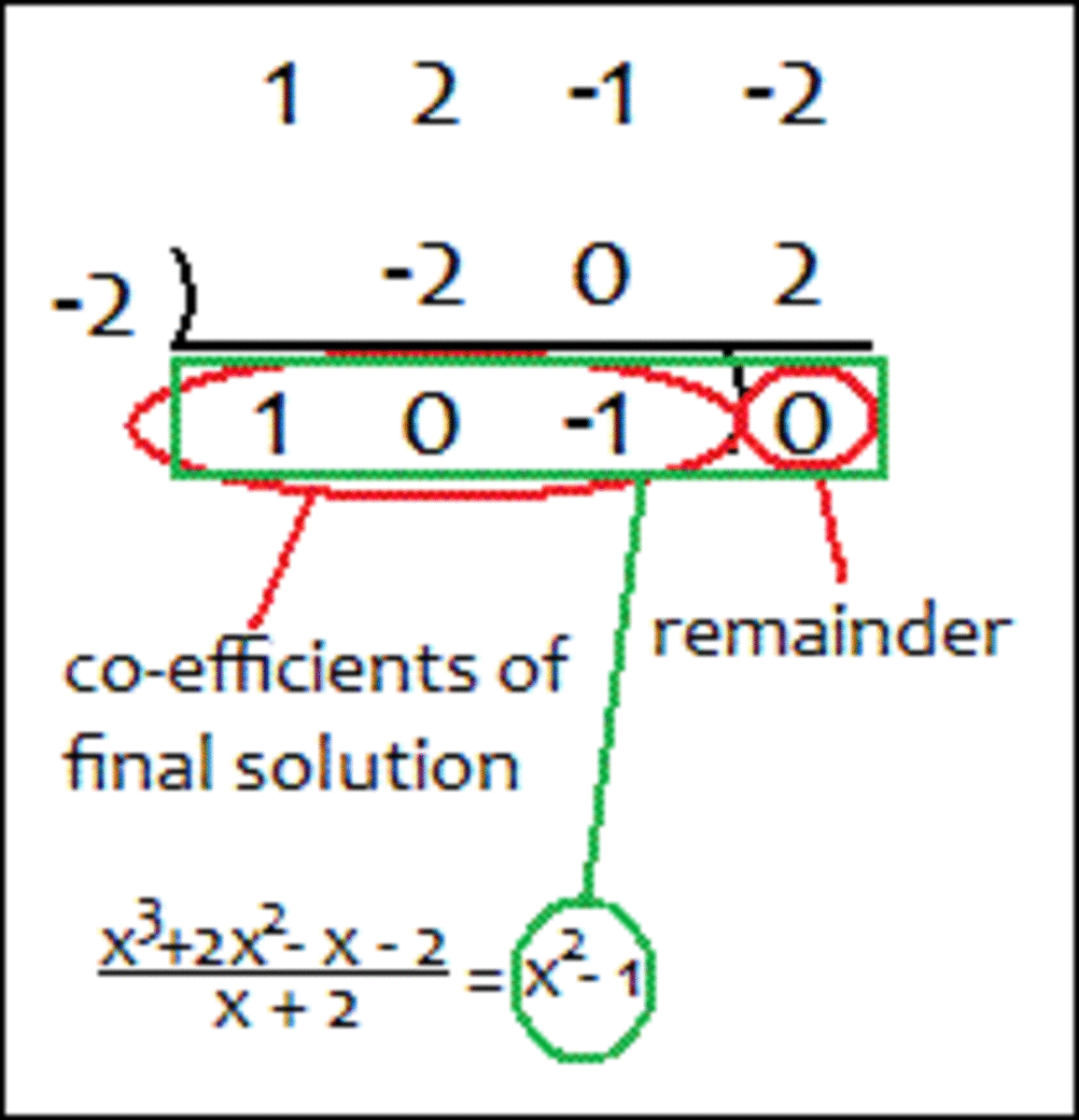# Math Help: How to Do Long Division of Polynomials Easily (Synthetic Division)

Stuck on long division of polynomials? The traditional long division method not doing it for you? Here's an alternative method which is possibly even easier and totally accurate—synthetic division.

This method can help you not only to solve long division equations, but to help you in turn to factorize polynomials and even solve them. Here is a simple, step-by-step guide to synthetic division.

## 1. What Is a Long Division Equation?

Firstly, you should probably be able to recognize what is meant by a long division equation. Here are some examples:

## 2. The Important Parts of Your Equation

Next, you need to be able to recognize within your equation a few key parts.

First, there is the polynomial you want to divide. Then, there are the co-efficients of the powers of x in the polynomial (x4, x3, x2, x, etc).* Finally, you should see what one solution of your equation is (e.g. if you are dividing by [x + 5], the solution is -5. As a general rule, if you are dividing the polynomial by [x-a], the solution is a).

*Note that any constant terms count as co-efficients - as they are co-efficients of x0. Also, keep in mind any powers of x that are missing and note that they have co-efficients of 0 - e.g. in the polynomial x2 - 2, the co-efficient of x is 0.

## 3. Setting Up Synthetic Division

Now, time to actually do the long division, using the synthetic division method. Here is an example of what your working should look like, including placement of co-efficients, the given solution, and your own solution, including the remainder.

(Note: we are continuing to use the example in the previous step.)What synthetic division looks like, and where to place certain parts of the equation and your working around the fancy line.

## 4. Adding the Numbers in Each Column

The next few steps are ones you repeat per "column" - as labelled in the diagram below.

The first of these repeated steps is to add the numbers in the column you are dealing with (you start with the first column on the left, then work right), and write the answer in the column below the line. For the first column, you simply write the first co-efficient below the line, as there is no number below it that needs to be added.

In later columns, when a number is written below the co-efficient (which is explained in step 5 below), you add up the two numbers in the column, and write the sum below the line, as you did for the first column.

## 5. Multiplying Numbers Below the Line by the Given Solution, then Placing the Answer in the Next Column

Here is the second step, step 5, to repeat for each column, after step 4 has been completed for the previous column.

Scroll to Continue

Once the first column is completed, you then multiply the number below the line in this column by the given solution on the left (labelled in step 3 above). As the title of this step suggests, you then write the solution to this calculation in the next column, underneath the co-efficient.

Remember: as step 4 above explains, you then add the two numbers in the column, and write the answer below the line. This gives you another number below the line to repeat this step 5. You repeat steps 4 and 5 until all columns have been filled out.

## 6. Recognizing the Final Solution and the Remainder

As labelled in the diagram below, all the numbers you have worked out and written under the line are the co-efficients of your final solution. The final number (in the last column), which you have separated from the rest with a curved line, is the remainder of the equation.

## 7. Writing Out Your Final Solution!

You know what the co-efficients of your final solution are. Just note that the final solution is of one degree less than the polynomial you just divided - i.e. if the highest power of x in the original polynomial is 5 (x5), the highest power of x in your final solution will be one less than that: 4 (x4).

Therefore, if the co-efficients of your final solution are 3, 0, and -1 (ignore the remainder), your final solution (ignoring the remainder for now) is 3x2 + 0x - 1 (i.e. 3x2 - 1).

Now, for the remainder. If the number in the final column is simply 0, there is, naturally, no remainder to the solution and you can leave your answer as is. However, if you have a remainder of, say, 3, you add on to your answer: + 3/(original polynomial). e.g. If the original polynomial you have divided is x4 + x2 - 5, and the remainder is -12, you add -12/(x4 + x2 - 5) to the end of your answer.Final solution to the division equation (co-efficient of x is 0, remainder is 0)

And there you have it, synthetic division! 7 steps seems like a lot, but they are all relatively short and there simply to make things absolutely, crystal clear. Once you get the hang of doing this process on your own (which should be after just a few goes), it is very quick and easy to use as working in exams and tests.

Some other uses of this method, as previously mentioned, include part of factoring a polynomial. For example, if one factor has already been found (perhaps by the factor theorem), then doing synthetic division of the polynomial, divided by this factor, can simplify the it down to the one factor multiplied by a simpler polynomial - which in turn may be easier to factorize.

Here's what this means: e.g. In the example used in the steps above, a factor of the polynomial x3 + 2x2 - x - 2 is (x + 2). When the polynomial is divided by this factor, we get x2 - 1. By the difference of two squares, we can see that x2 - 1 = (x + 1)(x - 1). Thus, the entire polynomial factorized reads: x3 + 2x2 - x - 2 = (x + 2)(x + 1)(x - 1).

To take this all a step further, this can help you to solve the polynomial. Thus, in the example used, the solution is x = -2, x = -1, x = 1.

Hopefully this has helped a little and you are now more confident in solving division problems involving polynomials.

annonymus on May 25, 2018:

great detailed explanation .only step 4 was a bit incomprehensible

thank you soo much

Donna Cosmato from USA on January 10, 2012:

Congratulations on being selected as a Hubnuggets nominee! I wish you had been my math teacher; I'm sure I would have understood the subject matter better and received a higher grade. Voted up.

Michelle Simtoco from Cebu, Philippines on January 07, 2012:

Gosh you are bringing me back to my high school and college when we needed to study this. This is truly helpful for students and this is a good idea for a hub! Keep up the great work.

Congratulations on your Hubnuggets nomination. Polynomials are in see for yourself https://enellelamb.hubpages.com/hubnuggets6/hub/Hu... be sure to read and vote and join the Hubnuggets fun! Have an amazing year ahead of you! Love and light.

Raj Lally Batala from Chicago ,USA on December 05, 2011:

good article !!

samanthamayer (author) from New Zealand on December 02, 2011:

Thank you for your comments! I must post more tutorial hubs, as I enjoy it and hope that they do manage to help some people.

Felixedet2000 from The Universe on December 02, 2011:

You are a teacher for real: keep it up, love your hub.

sestasik on December 02, 2011:

This is a great math hub with some serious work put in. Would really be helpful for someone looking for homework or study help. Good job and voted up!